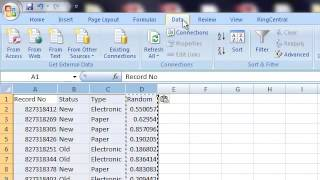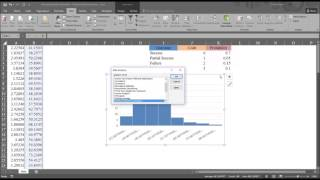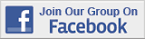Excel Tips & Tutorials:
Random Assignment and Sampling
 Suggest a Link Alphabetize Page Printer-Friendly List
Watch and Learn
Watch and Learn
Watch and Learn
Watch and Learn
 How to Create A Stratified Random SampleFrom Tim D'Auria (5:25)How to Create Simple Random Sample and Systematic SampleFrom Farhad Zulfiqar (5:18)Random Number Generation with Multiple DistributionsDistributions reviewed include uniform, normal, Bernoulli, binomial, Poisson, patterned, and discrete (11:36).Efficient Data Entry wih Excel FormsLeila Gharani demonstrates (6:21).How to Create A Stratified Random SampleFrom Tim D'Auria (5:25)How to Create Simple Random Sample and Systematic SampleFrom Farhad Zulfiqar (5:18)Random Number Generation with Multiple DistributionsDistributions reviewed include uniform, normal, Bernoulli, binomial, Poisson, patterned, and discrete (11:36).How to Create A Stratified Random SampleFrom Tim D'Auria (5:25)How to Create Simple Random Sample and Systematic SampleFrom Farhad Zulfiqar (5:18)How to Create A Stratified Random SampleFrom Tim D'Auria (5:25)Sampling with Excel Short how-to article from Real Statistics Using Excel web site.Sampling with Excel Short how-to article from Real Statistics Using Excel web site.Sampling with Excel Short how-to article from Real Statistics Using Excel web site.Sampling with Excel Short how-to article from Real Statistics Using Excel web site.Over 18,000 Members!